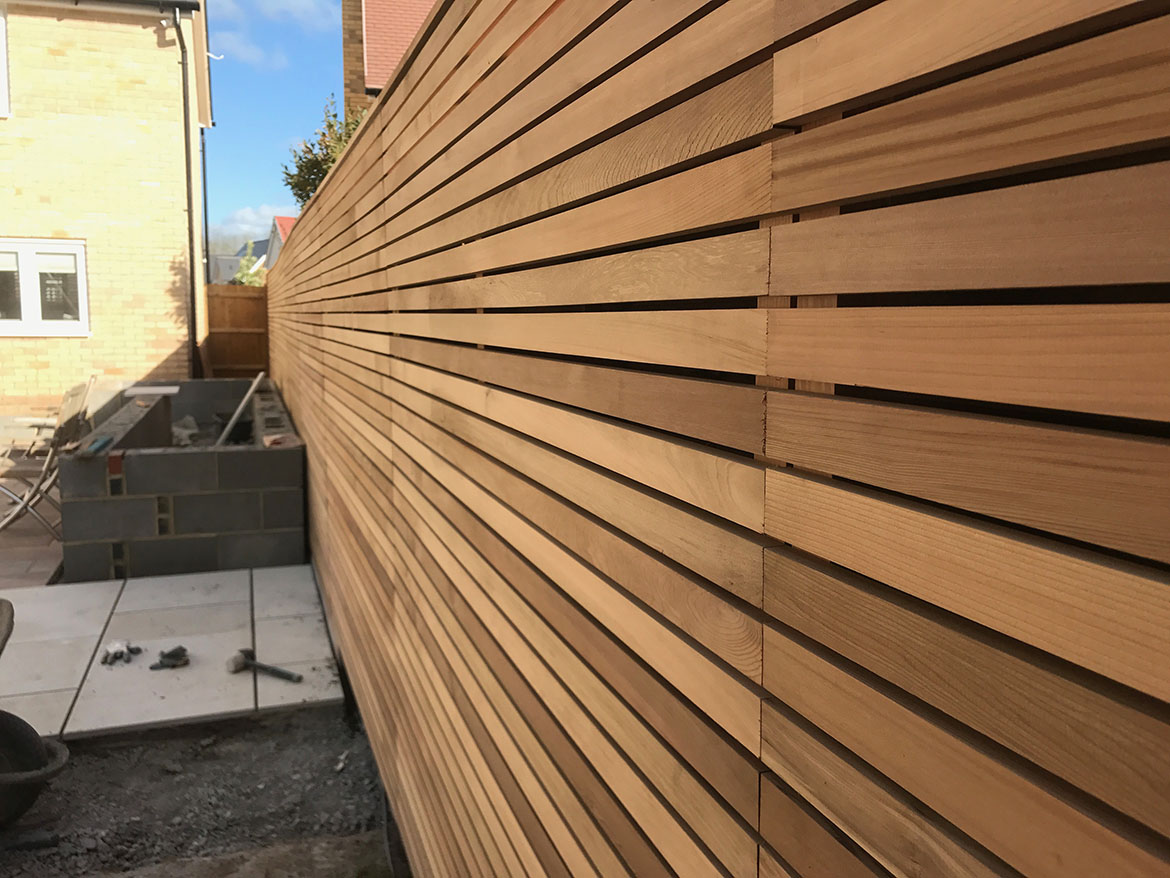Uncategorized

•Example Blog Post

This is where your content would go. This is where your content would go. This is where your content would go. This is where your content would go. This is where your content would go. This is where your content would go. This is where your content would go. This is where your content would go. This is where your content would [...]

By | 2017-05-17T09:05:14+00:00 May 17th, 2017|Uncategorized|0 Comments